Home Practice
For learners and parents For teachers and schools
Textbooks
Full catalogue
Pricing SupportLog in

We think you are located in United States. Is this correct?

# 8.2 Personal income and expenditure

## 8.2 Personal income and expenditure (EMG5C)

### Income (EMG5D)

An income is any way in which people earn money, and there are many different types of income to consider. Here are some of the ways in which people earn money.

• Some people have jobs and receive wages or salaries.
Salary
A fixed amount of money paid by an employer to an employee for work done over some longer period of time - usually per month or per year.
Wage
An amount of money paid by an employer to an employee for work done in some shorter period of time - usually hours or days per week.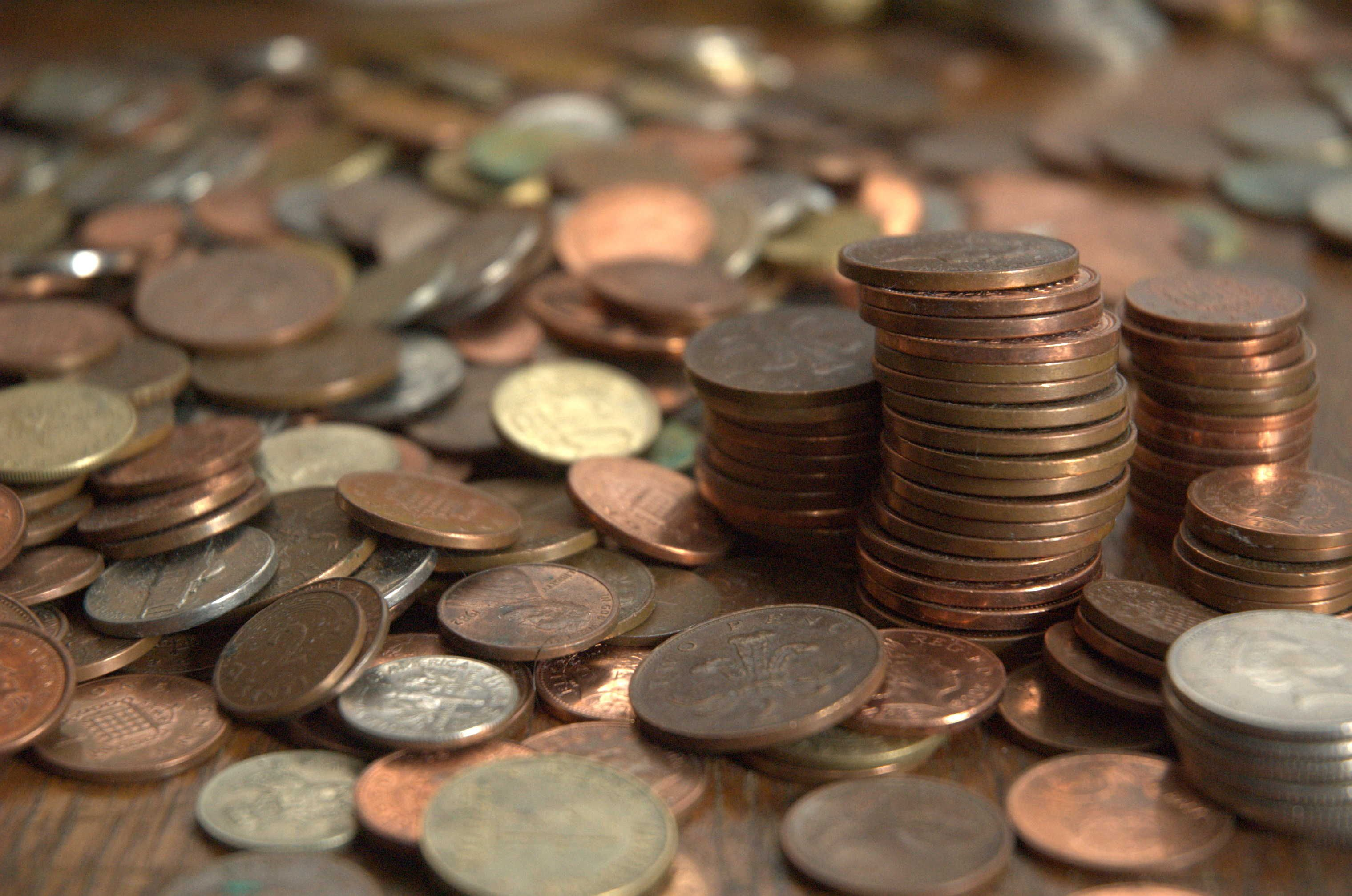• Some people may earn commission, which is a fixed, extra amount of money paid to them, based on an agreement for providing additional services. For example, a hairdresser might earn a basic salary for doing hairdressing, but could earn extra money (a commission) for also selling the salon's products, such as shampoos and conditioners, to customers.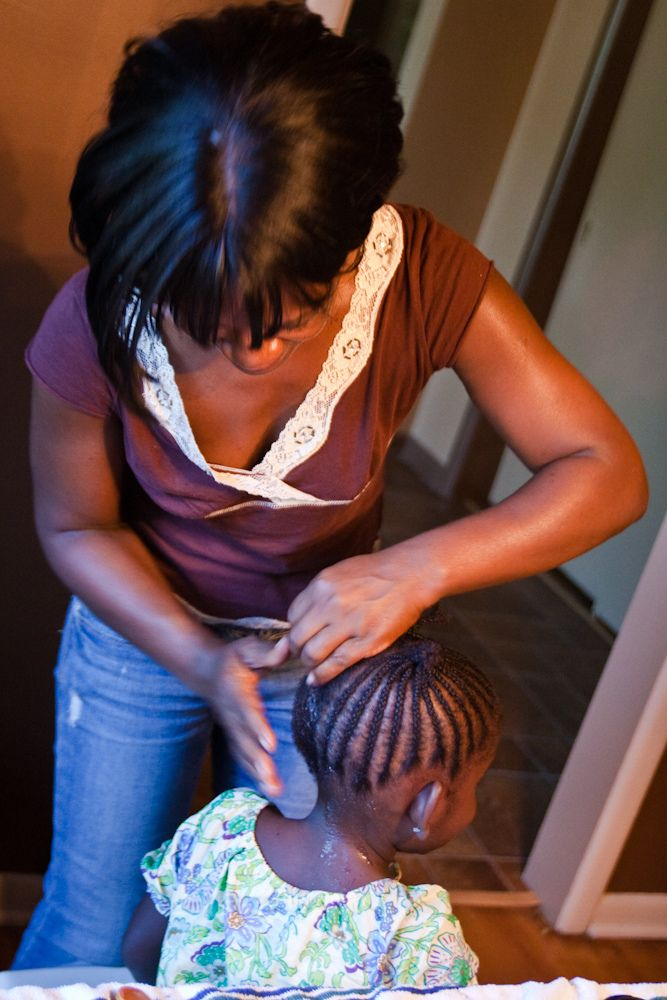• If you produce and sell goods or services, the money you make - in the form of profit - is also income. To calculate your profit on any sale, you need to subtract the cost of producing the goods or providing the services from the total amount of income you received.
• People working in the service industry could also receive gratuities (tips) as well as their wages.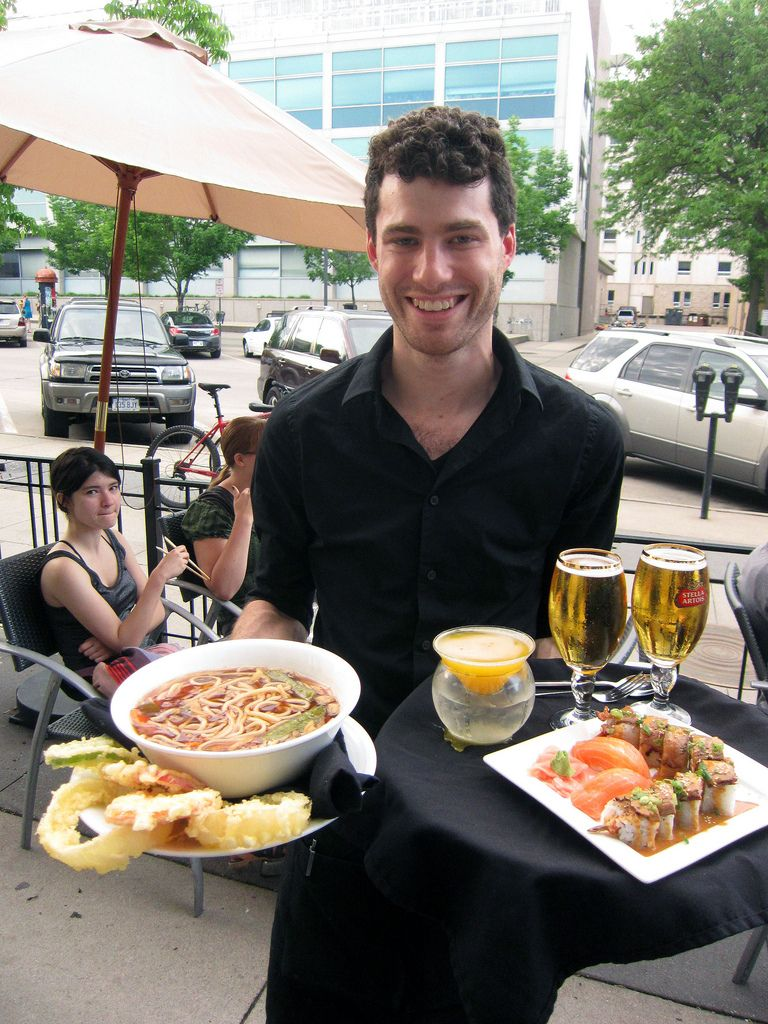• People sometimes receive money as a gift, and young people sometimes receive pocket money from their parents or guardians.
• Someone who owns a property could rent it out and receive rental income.
Budget
A budget is a plan of action to balance your expenditure with your income. it is usually displayed as a table of income items in one column and expenditure items in another. The last row of the budget shows the difference between income and expenditure. When income exceeds expenditure (your income is more than your expenses) then it is called a surplus. when expenditure exceeds income (your expenses are more than your income) then it is called a deficit or shortfall.
Study loan
A loan granted to students to assist them with all financial matters throughout their study period. it is a loan that is only given providing certain conditions are met which probably include (after graduation) a repayment plan.
Bursary
A bursary is financial assistance that is granted to previously disadvantaged students. bursaries are mostly granted by government, as well as by large companies in the private sector.

Personal income is money that is paid to you, or that comes into your possession or bank account. Income can be paid to you in the form of a salary or wage for work undertaken, and it ranges from gifts or pocket money (e.g. from your parents) to bursaries or loans, savings, interest earned on your savings and income from inheritance.

There are three types of income: fixed, variable and occasional income.

• Fixed income is an amount of money a person receives, which does not change with time. Salaries and wages are examples of fixed income.
• Variable income is an amount of money a person receives that changes over time, or changes according to the situation. Commissions and interest on investments or savings are examples of variable income.
• Occasional income is when someone receives money from time to time. Examples of occasional income may include gifts (e.g. money for your birthday) or inheritance (e.g. if a family member passes away and leaves money to you in their will).

## Worked example 1: Classifying personal income

Mr Sibong works as a sales manager for his company. He is a full-time employee, and he also gets commision on all sales he makes, on behalf of the company.The table below lists the different types of income he receives.Study the table and place a tick in one of the boxes for each row, to indicate whether the type of income is fixed, variable or occasional.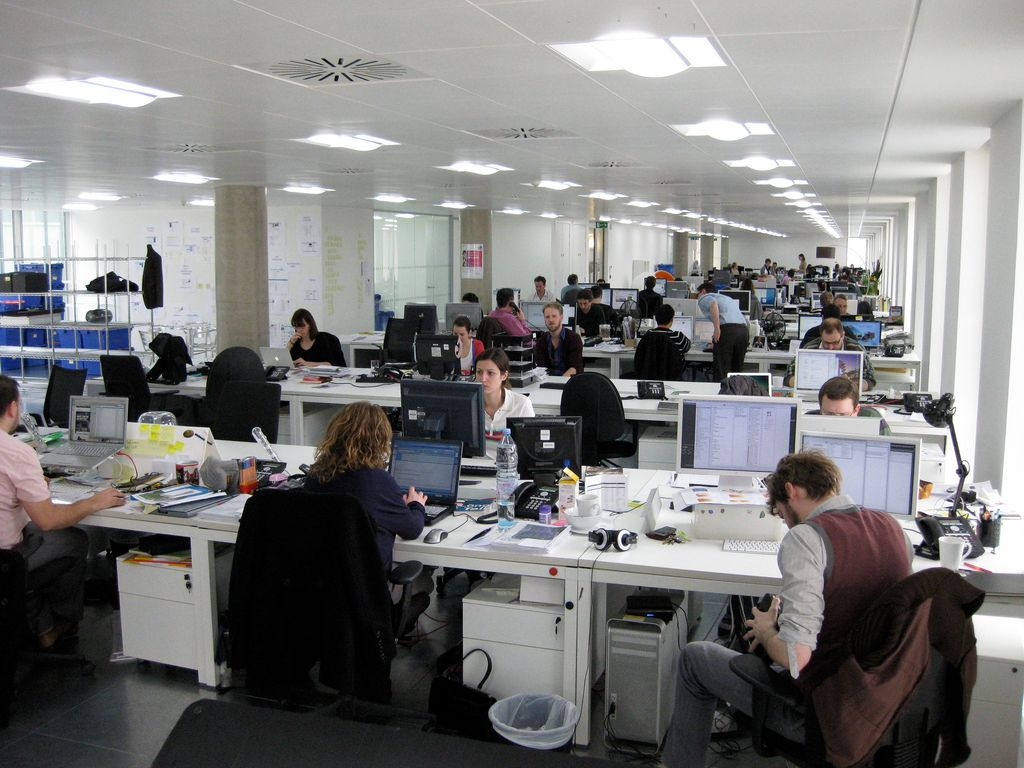Types of income Fixed Variable Occasional Salary Interest received from fixed account Income received from renting out a flat Bonus Cash gift from friends Sales commission Transport allowance
 Types of income Fixed Variable Occasional Salary Fixed Interest received from fixed account Fixed Income received from renting out a flat Fixed Bonus Occasional Cash gift from friends Occasional Sales commission Variable Transport allowance Fixed

## Personal income

Exercise 8.1

Read the paragraph below and identify all Petrus's sources of income. Classify each source of income as fixed, variable or occasional. Petrus has just started his first job and he earns a basic salary as a sales representative, and also receives allowances for cell phone and travel. He also gets paid commission every three months on the sales that he makes. He has started a small music band and he sometimes gets asked to play at events such as birthday parties and weddings, where he negotiates his hourly fee.Fixed: basic salary, allowances for cell phone and travel. Variable: commission. Occasional: hourly fee for band performances.

You are currently in Grade 10 and in order to earn extra money you accept a job at a Spur restaurant as a waiter. You work the following shifts per month:

• Four Friday shifts per month for $$\text{5}$$ hours. Friday rate/hour = $$\text{R}\,\text{20}$$
• Four Saturday shifts per month for $$\text{10}$$ hours. Saturday rate/hour = $$\text{R}\,\text{30}$$
• Two Sunday shifts per month for $$\text{8}$$ hours. Sunday rate/hour = $$\text{R}\,\text{40}$$
• Estimated tips earned per month = $$\text{1,5}$$ $$\times$$ your monthly salary.

Calculate your total income for one month.

Total Income earned = [($$\text{4}$$ $$\times$$ $$\text{5}$$) $$\times$$ $$\text{R}\,\text{20}$$ + ($$\text{4}$$ $$\times$$ $$\text{10}$$) $$\times$$ $$\text{R}\,\text{30}$$ + ($$\text{2}$$ $$\times$$ $$\text{8}$$) $$\times$$ $$\text{R}\,\text{40}$$ ] = [$$\text{R}\,\text{400}$$ + $$\text{R}\,\text{1 200}$$ + $$\text{R}\,\text{640}$$] = $$\text{R}\,\text{2 240}$$ / month

### Expenditure (EMG5F)

Personal expenditure is money that you spend. Expenditure can include living expenses (e.g. food, clothing, entertainment), accounts (e.g. water, electricity, telephone), fees (e.g. school fees), insurance (e.g. for a car or house), taxes and loan repayments (e.g. to pay off your store account).

As with expenditure, there are fixed, variable and occasional or unexpected expenses. Fixed expenses are the amounts of money a person spends, which do not change over time - for example rental for a flat. Variable expenses are amounts of money a person spends that do change over time. Groceries or electricity bills are examples of variable expenses. Occasional or unexpected expenses are sometimes expenses that you cannot plan for, for example a visit to the doctor, or repairs to your car if it breaks down. Some occasional expenses can be planned for, for example, annual car services.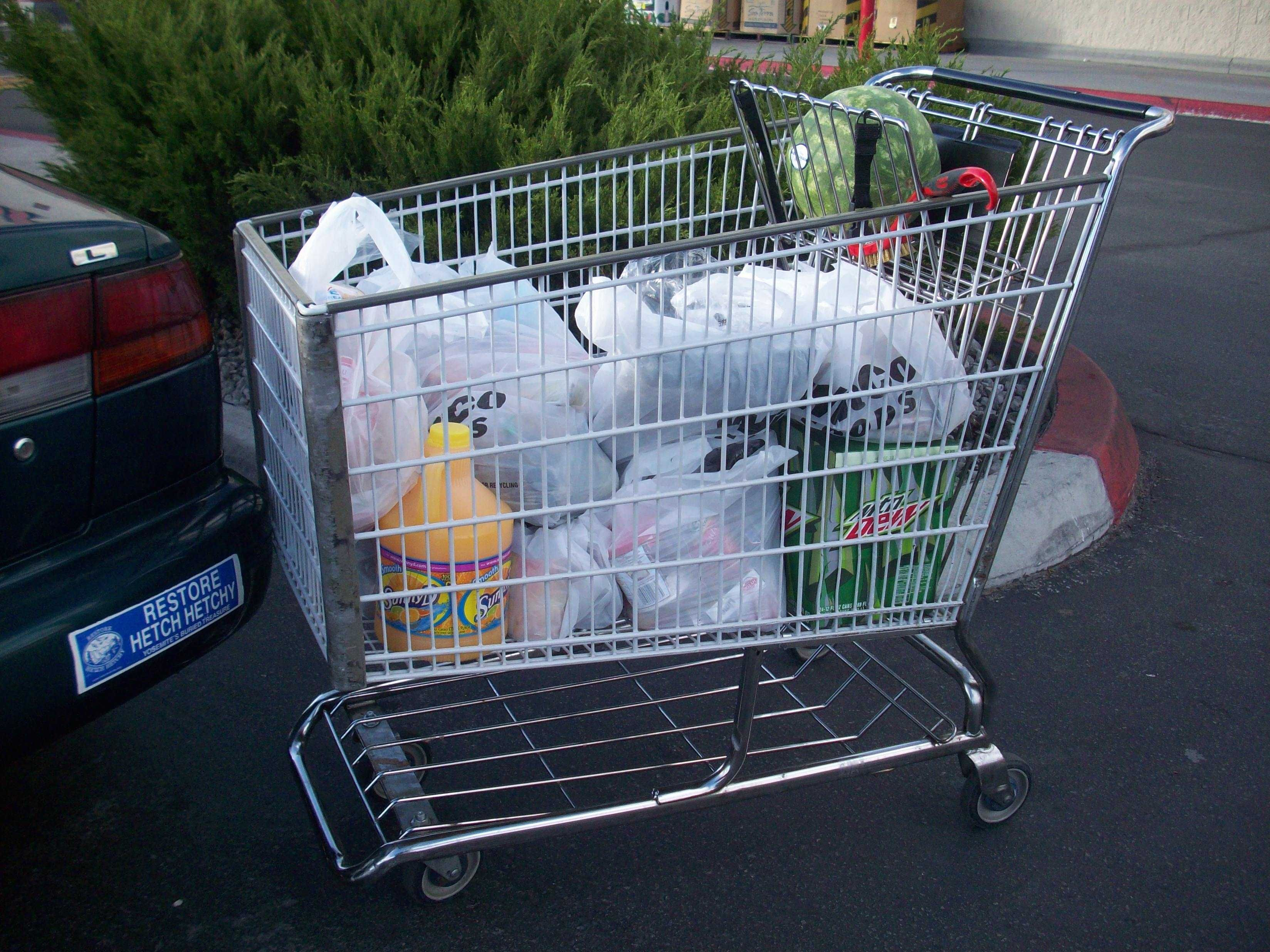It is important to monitor your income and expenses each month, in order to manage your money carefully and plan your activities in such a way that you do not spend more money than you have, and get into debt. It is also important to try to save money so that you can pay for unexpected expenses (like a visit to the doctor). You can also decide which of your expenses are high and low priority. For example, if you are running low on money at the end of a month, buying food is more important, or of higher priority, than buying music CDs or going to the movies.

Debt
The amount of money owed.

## Worked example 2: Classifying personal expenditure

Complete the table below by classifying the following expenses as fixed, variable or occasional:

 Types of expenses Fixed Occasional Variable Rent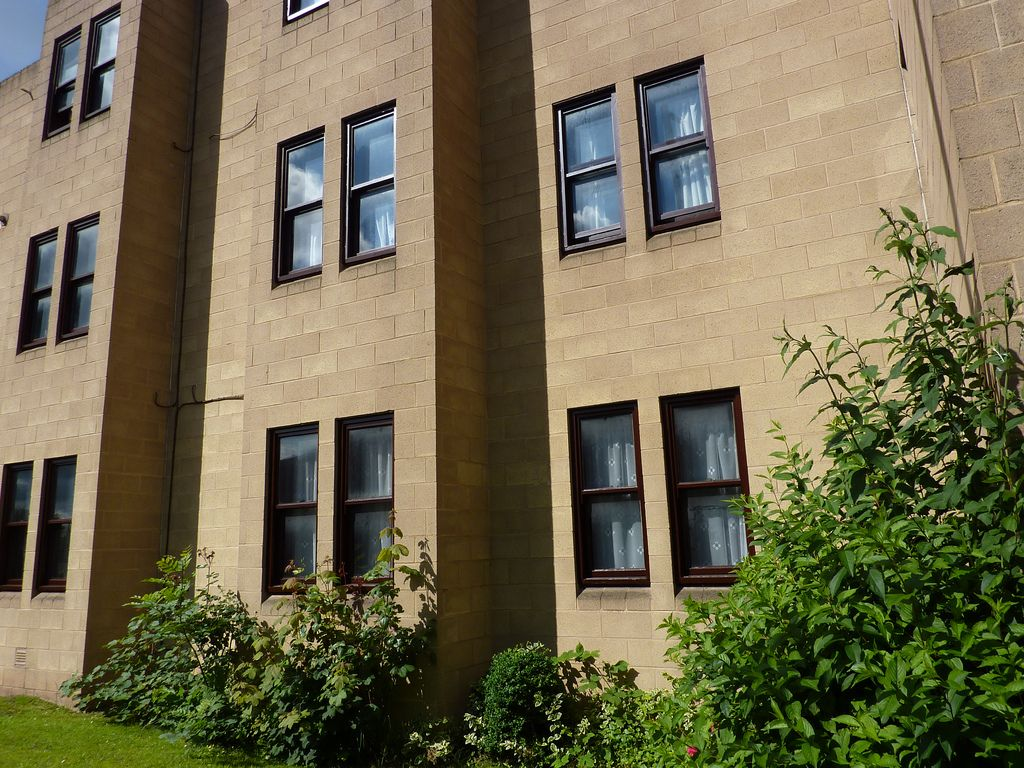Transport (public) Groceries Rates and taxes Vehicle repairsTelkom account Vodacom contract account Water and electricity Petrol Insurance premium
 Types of expenses Fixed Occasional Variable Rent Fixed Transport (public) Variable Groceries Variable Rates and taxes Fixed Vehicle repairs Occasional Telkom account Variable Vodacom contract account Fixed Water and electricity Variable Petrol Variable Insurance premium Fixed

## Personal expenditure

Exercise 8.2

You decide to spend your income in the proportions shown below:

 Clothes $$\text{30}\%$$ Entertainment $$\text{10}\%$$ Savings $$\text{10}\%$$ Charitable organisations $$\text{25}\%$$ Transport $$\text{12}\%$$ Sweets and cool drinks$$\text{13}\%$$ Total: $$\text{100}\%$$

If you earn an income of $$\text{R}\,\text{1 200}$$ in a particular month, calculate exactly how much money you will be spending on each of the above items.

 Clothes $$\text{30}\%$$ $$\times$$ $$\text{R}\,\text{1 200}$$ = $$\text{0,30}$$ $$\times$$ $$\text{R}\,\text{1 200}$$ = $$\text{R}\,\text{360}$$ Entertainment $$\text{10}\%$$ $$\times$$ $$\text{R}\,\text{1 200}$$ = $$\text{0,10}$$ $$\times$$ $$\text{R}\,\text{1 200}$$ = $$\text{R}\,\text{120}$$ Savings $$\text{10}\%$$ $$\times$$ $$\text{R}\,\text{1 200}$$ = $$\text{0,10}$$ $$\times$$ $$\text{R}\,\text{1 200}$$ = $$\text{R}\,\text{120}$$ Charitable organisations $$\text{25}\%$$ $$\times$$ $$\text{R}\,\text{1 200}$$ = $$\text{0,25}$$ $$\times$$ $$\text{R}\,\text{1 200}$$ = $$\text{R}\,\text{300}$$ Transport $$\text{12}\%$$ $$\times$$ $$\text{R}\,\text{1 200}$$ = $$\text{0,12}$$ $$\times$$ $$\text{R}\,\text{1 200}$$ = $$\text{R}\,\text{144}$$ Sweets and cool drinks $$\text{13}\%$$ $$\times$$ $$\text{R}\,\text{1 200}$$ = $$\text{0,13}$$ $$\times$$ $$\text{R}\,\text{1 200}$$ = $$\text{R}\,\text{156}$$ Total: $$\text{100}\%$$ = $$\text{R}\,\text{1 200}$$